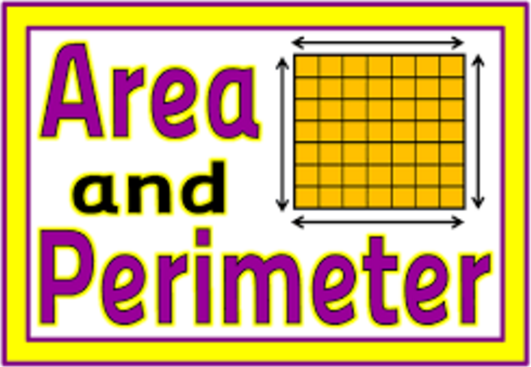Area and Perimeter
4 years ago
jsolnek
Save
Edit
Host a game
Live GameLive
Homework
Solo Practice
Practice12 QuestionsShow answers
• Question 1
300 secondsQ. What is the perimeter of this figure?
42 cm
49 cm
56 cm
63 cm
• Question 2
300 seconds
Q. The length of one side of a square is 9 inches. What is the perimeter? (Hint: P=4s)
81 inches
36 inches
27 inches
18 inches
• Question 3
300 seconds
Q. Mrs. Washington is putting up a wallpaper border around her son’s bedroom. The bedroom is the shape of a rectangle that is 18 feet wide by 19 feet long. How many feet of border will Mrs. Washington need? (Hint: P = 2l + 2w)
37 feet
72 feet
74 feet
76 feet
• Question 4
300 seconds
Q. The perimeter of a parallelogram is 16 meters. One short side is 2 meters long. What is the length of one long side of the parallelogram?
2 meters
6 meters
18 meters
36 meters
• Question 5
300 secondsQ. Coach Hanks has his players run laps around the soccer field. What is the perimeter of the soccer field shown below? (Hint: P = 2l + 2w)
185 m
250 m
370 m
380 m
• Question 6
300 seconds
Q. Nick’s rectangular patio measures 9 feet wide and 12 feet long. What is the perimeter of the patio?
21 feet
42 feet
54 feet
108 feet
• Question 7
300 secondsQ. What is the area of the figure?
22 square feet
44 square feet
98 square feet
105 square feet
• Question 8
300 seconds
Q. What is the area of a rectangle with a length of 15 m and a width of 5 m?
40 square meters
50 square meters
75 square meters
100 square meters
• Question 9
30 seconds
Q. The perimeter of a square is 24 centimeters. What is the area of the square?
24 square centimeters
36 square centimeters
37 square centimeters
576 square centimeters
• Question 10
300 secondsQ. The perimeter of the rectangle shown below is 28 centimeters. What is the area of the rectangle?
12 square centimeters
14 square centimeters
36 square centimeters
48 square centimeters
• Question 11
300 secondsQ. Find the length of the rectangle.
4 mi
8 mi
21 mi
30 mi
• Question 12
300 seconds
Q. Nassim wants to build a kitchen table with a top that has an area of 20 square feet. Which dimensions make the most sense for the table?